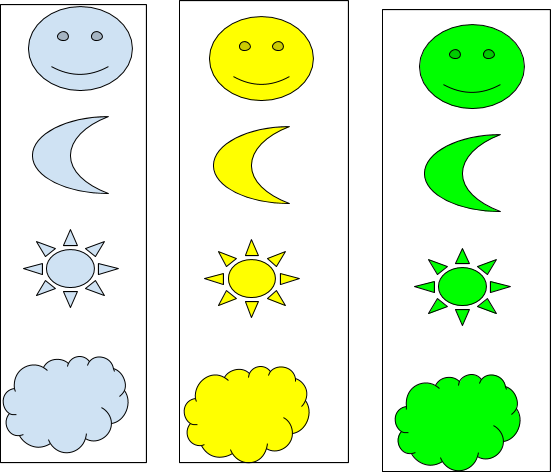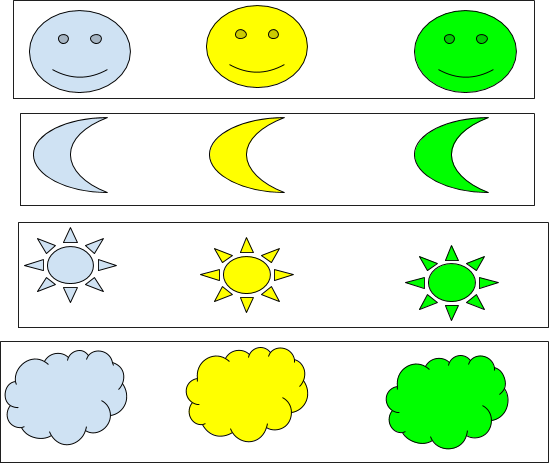$$\newcommand{\id}{\mathrm{id}}$$ $$\newcommand{\Span}{\mathrm{span}}$$ $$\newcommand{\kernel}{\mathrm{null}\,}$$ $$\newcommand{\range}{\mathrm{range}\,}$$ $$\newcommand{\RealPart}{\mathrm{Re}}$$ $$\newcommand{\ImaginaryPart}{\mathrm{Im}}$$ $$\newcommand{\Argument}{\mathrm{Arg}}$$ $$\newcommand{\norm}{\| #1 \|}$$ $$\newcommand{\inner}{\langle #1, #2 \rangle}$$ $$\newcommand{\Span}{\mathrm{span}}$$

# 2.2 Equivalence Relations, and Partial order

•• Contributed by Pamini Thangarajah
• Associate Professor (Mathematics & Computing) at Mount Royal University

$$\newcommand{\vecs}{\overset { \rightharpoonup} {\mathbf{#1}} }$$

$$\newcommand{\vecd}{\overset{-\!-\!\rightharpoonup}{\vphantom{a}\smash {#1}}}$$

Definition

An equivalence relation on a non-empty set $$S$$ is a relation that is reflexive(R), symmetric(S) and transitive(T).

Example $$\PageIndex{1}$$: $$=$$

Definition

Given an equivalence relation $$R$$ over a set $$S,$$ for any $$a \in S$$ the equivalence class of a is the set $$[a]_R =\{ b \in S \mid a R b \}$$, that is
$$[a]_R$$ is the set of all elements of S that are related to $$a$$.

Example $$\PageIndex{2}$$:

Define a relation that two shapes are related iff they are the same color. Is this relation an equivalence relation?

Equivalence classes are:## Example $$\PageIndex{3}$$:

Define a relation that two shapes are related iff they are similar. Is this relation an equivalence relation?

Equivalence classes are:Theorem:

If $$R$$ is an equivalence relation over a non-empty set $$S$$, then every $$a \in S$$ belongs to exactly one equivalence class.

Theorem $$\PageIndex{1}$$

If $$R$$ is an equivalence relation over a non-empty set $$S$$. Then

Then the set of all equivalence classes is denoted by $$\{[a]| a \in S\}$$ forms a partition of $$S$$.

This means

1. Either $$[a] \cap [b] = \emptyset$$ or $$[a]=[b]$$, for all $$a,b\in S$$.

2. $$S= \cup_{a \in S} [a]$$.

Proof

Add proof here and it will automatically be hidden if you have a "AutoNum" template active on the page.

Note

For every equivalence relations over $$S$$, $$S$$ has a partition.

Definition

A binary relation is a partial order if and only if the relation is reflexive(R), antisymmetric(A) and transitive(T).

Example $$\PageIndex{4}$$: $$\leq$$

Definition

Hasse diagram